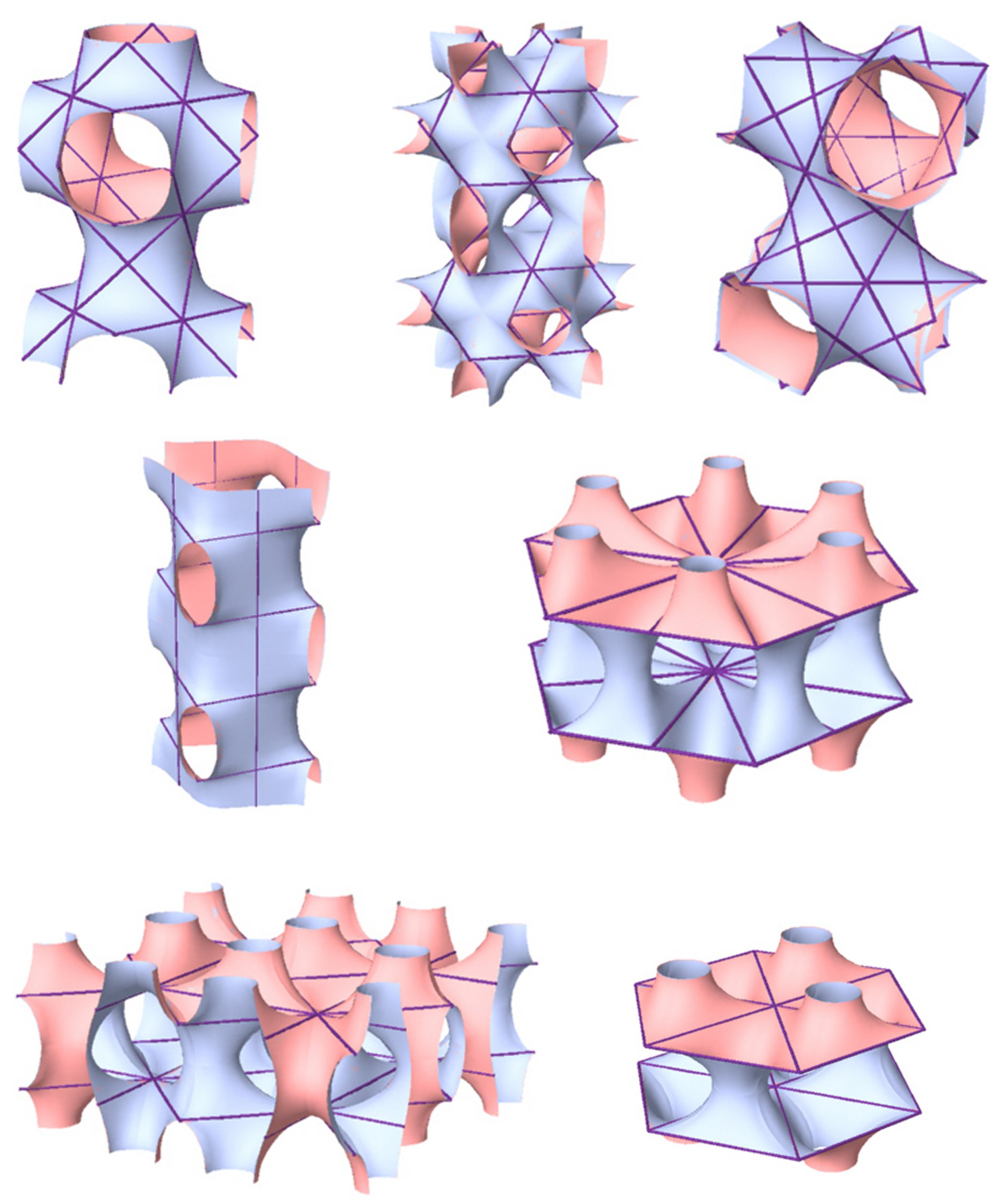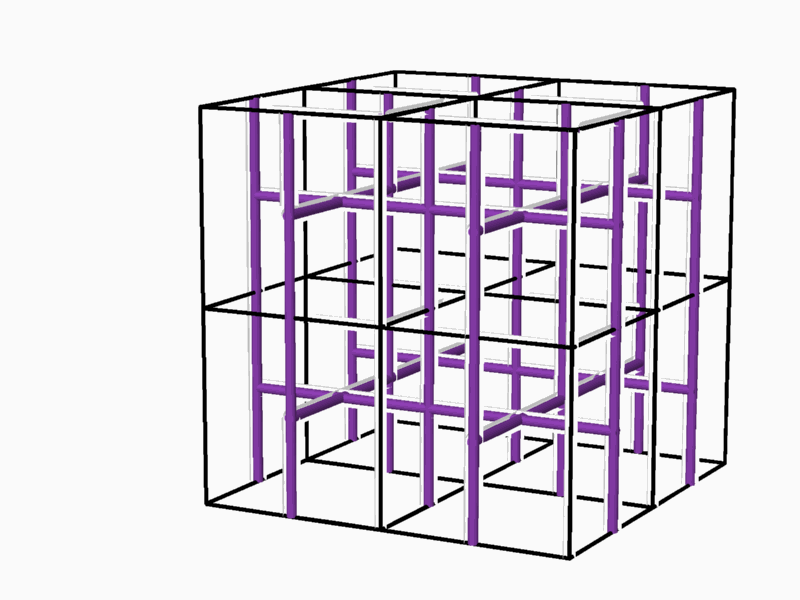2-manifold surfaces and space partitions By Ph.D.  Ami Korren Faculty of Architecture & Town Planning,  Technion.  Israel Institute of Technology,  Technion City, Haifa 32000, Israel.  E-mail: Korren-a@inter.net.il Abstract  The issue of space partitioning underlies the architectural planning and design of buildings, structures and spaces intended for human activity. This thesis explores the phenomenon of periodic dual spaces, the periodic three-dimensional networks that represent their inner structure and the partition between them.  It is assumed that every dual-pair of networks, (often referred to as complementary or reciprocal pairs), (Figure 1) can be separated and partitioned by a continuous smooth hyperbolic surface.Figure 1 - two dual networks Duality relation between the two lattices, meaning: 1:1 relation between edges of one of the lattices to the faces of the dual. 1:1 relation between vertices of one of the lattices to the packing cells of the dual, and vice versa. The thesis is focused on the unique phenomenon of identical dual spaces and the related network pairs, and the hyperbolic surface-partitions separating them, and thus dividing the entire space into two identical (complementary) sub-spaces (Figure 2).  The adopted approach implies investigation of order and organization of these spaces and related parameters, their inner and overall symmetry structure and the nature of the 2-manifold partitions, dividing between them.Figure 2 An additional central goal was to conduct a systematic exhaustive search of possible (thus defined) surface-partitions, in order to establish their range of existence and to facilitate their classification. The study described in this thesis comprises three stages.      1 Topological attributes of smooth 2-manifold which  divide the space into two identical subspaces.   The first stage consisted of studying identical dual spaces, their properties and parameters trying to develop insights into their nature and order.Figure 3 The tunnel-like periodic spaces, represented by networks, are defined within the Euclidean three-dimensional space, and are composed of periodic cells. This property indicates the relation between the network-pairs,| and the surface separating them, and the symmetry groups acting on this space.  The smallest repetitive cell, called "Elementary Periodic Region" (E.P.R.) (Figure 3), is derived from a periodical space using the symmetry operations of the symmetry group that acts on this space. The E.P.R. contains complete representation of all the phenomena taking place within the "periodic complex" and particularly, representation of the whole periodic space, its symmetry group, the two complementary (identical) subspaces, the self dual lattice-pairs characterizing them, as well as the surface partition in between.  At this stage of the research, the topological properties of smooth 2-manifolds in general, and of smooth 2-mainfolds which divide the space between two dual networks in particular, were explored.  The main properties of these 2-manifolds are (Figure 3):         * They are smooth, periodical and hyperbolic, and exist in the three-dimensional space.         * They divide the space into two identical subspaces that graphically represent two interwoven and             non-intersecting tunnel-networks. The two complementary tunnel systems are identical in volume             and shape, and their tunnel axes form two identical, three-dimensional space networks.         * 2-fold rotation axes contained within the 2-manifold rotate one subspace into the identical complimentary             subspace, forming in it a periodic (three-dimensional) space network,referred to as a "2-fold network".Figure 4 2 The method of searching and classifying of the 2-manifold. In the second stage, a method for the enumeration and classification of the 2-manifolds that divide the space into two identical subspaces and identification of the axes of the identical dual tunnel networks was developed. The method of enumerating the 2-manifolds was based on their topological properties that were studied in the previous stage.  The issues of periodic minimal hyperbolic 2-manifolds that divide the space into two identical subspaces were investigated in the past. Seven surfaces were found (Figure 4).Figure 5 It was clear even then that numerous identical networks and a smooth hyperbolic partition dividing them could be put through every E.P.R. containing 2-fold axes. No attempt has been made to date either to construct or characterize these surfaces or to define the range of their existence and to provide their classification.  The existence and periodicity of the 2-manifolds that divide the space into two identical subspaces in the Euclidean three-dimensional space indicate the link between these 2-manifolds and the symmetry groups operating within this space.  The "atomistic" conception of space suggests the existence of an E.P.R. that represents all the properties of the complex. Finding all the E.P.R.s, which represent identical-dual spaces and the 2-manifold in between them, leads to discovery of the 2-manifolds.  The number of the different E.P.R.s is finite due to the fact that the number of symmetry groups, which operate in the Euclidean space is finite.  The method for the enumeration and classification of the 2-manifolds consists of several consecutive steps.           * Locating all the E.P.R.s derived from the symmetry groups (Figure 5).           * Reducing the list of the relevant E.P.R.s to those containing 2-fold axes, capable of rotating the E.P.R.                into itself (Figure 6).             *Obtaining the 2-fold networks by repetitive duplication of the E.P.R.s found in the previous stage (Figure 7).          * Locating periodic cells enclosed in the 2-fold networks (more than one is likely), (which may enclose a periodic              unit of the 2-manifold,) and conducting exhaustive search to the possible 2-manifold units (Figure 8).           * Duplicating the periodic cells with the enclosed periodic unit of the 2-manifolds, until a large enough section               of the surface is obtained, enabling identification of the self-dual tunnel networks (Figure 9).             * Topologically enumerating each of the 2-manifolds, according to their respective tunnel network.Figure 6 - E.P.R.s containing 2-fold axes, that rotate the E.P.R. into itselfFigure 7 - Two different 2-fold networksFigure 8  Two different periodic cells located in the same 2-fold networkFigure 9 3 The process of searching and classifying of the required 2-manifold. In the third stage, by applying the previously developed method, a process of the actual identification of the self-dual spaces was carried out.   Finding the 2-fold networks is supposed to be exhausted at this stage. The method of locating the 2-fold networks is based on identifying the E.P.R.s that may, as stated earlier, contain 2-fold axes that rotate them into themselves.  At this stage fourteen different "2-fold networks" were found. Among those, twelve were found to contain seventeen different closed periodic cells, which may enclose a periodic unit of a 2-manifold.  Duplicating these closed cells led, to the discovery of eight topologically different 2-manifolds. Among them, a new 2-manifold, unknown to date, was discovered. (Since then, yet another 2-manifold was discovered) (Figure 10).Figure 10 - A new 2-manifold that was discovered. Among the seventeen different closed periodic cells, mentioned earlier, there are six closed cells that consist of a "split perimeter". These "split perimeters" can enclose a "periodic 2-manifold unit" of different category surfaces, i.e. they can enclose in the periodic closed perimeter cell a successive smooth hyperbolic "periodic 2-manifold unit", which is topologically different from the minimal "periodic 2-manifold unit" previously discovered. Thus a new class of 2-manifolds, designated "The Multiple- Sleeved Class" was found (Figure 11). This class contains an infinite number of topologically different 2-manifolds. This indicates that the tunnel axes of these 2-manifolds represent pairs of identical-dual networks that differ from each other.Figure 11 The proposed method of identifying the 2-manifolds that divide between pairs of self-dual networks, led to the discovery of new 2-manifolds in addition to the seven 2-manifolds, of which two were first discovered by Hermann Amandus Schwarz (1843-1921). The 2-manifolds were looked for in representative groups, relating to symmetry groups, the number of which is finite. This does not necessarily mean that the number of 2-manifolds, and/or the number of self-dual pairs of networks are also finite.  References A. Korren, (2003) Identical Dual Lattices and Subdivision of Space. Ph.D. Thesis.                             Technion  Israeli Institute of Technology.